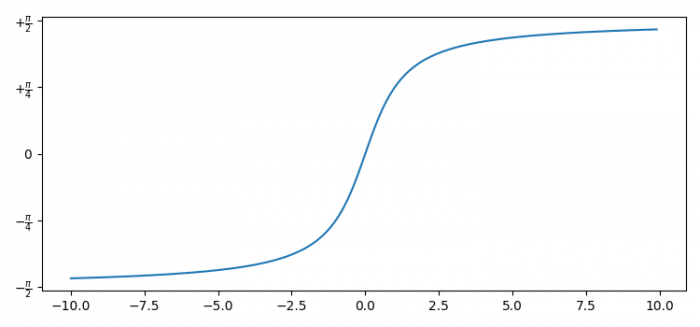# How to set the Y-axis in radians in a Python plot?

To set the Y-axis in radians in a Python plot, we can take the following steps −

• Set the figure size and adjust the padding between and around the subplots.
• Create x and y data point using numpy.
• Create a new figure or activate an existing figure using figure() method.
• Add an axes, ax, to the figure as part of a subplot arrangement.
• Get the list of Y-axis ticks and ticklabels.
• Set the ticks and ticklabels using set_yticks() and set_yticklabels() methods.
• To display the figure, use show() method.

## Example

import matplotlib.pyplot as plt
import numpy as np

plt.rcParams["figure.figsize"] = [7.50, 3.50]
plt.rcParams["figure.autolayout"] = True

x = np.arange(-10.0, 10.0, 0.1)
y = np.arctan(x)

fig = plt.figure()
ax.plot(x, y)
unit = 0.25

y_tick = np.arange(-0.5, 0.5 + unit, unit)
y_label = [r"$-\frac{\pi}{2}$", r"$-\frac{\pi}{4}$", r"$0$", r"$+\frac{\pi}{4}$", r"$+\frac{\pi}{2}$"]

ax.set_yticks(y_tick * np.pi)
ax.set_yticklabels(y_label, fontsize=10)

plt.show()

## OutputUpdated on: 03-Aug-2021

525 Views